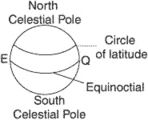# circle of latitude

(redirected from Circles of latitude)
Also found in: Dictionary.
Related to Circles of latitude: circle of longitude

## circle of latitude

(astronomy)
A great circle of the celestial sphere passing through the ecliptic poles, and hence perpendicular to the plane of the ecliptic. Also known as parallel of latitude.
(geodesy)
A meridian of the terrestrial sphere along which latitude is measured.

## circle of latitudei. A great circle of the celestial sphere through the ecliptic poles and, hence, perpendicular to the plane of the ecliptic.
ii. A meridian along which latitude is measured.
References in periodicals archive ?
Figure 5 or Quinlan's model should help students see that the longitudinal differences are the same across all circles of latitude.
Students should be asked to mark on the transparent model two points that are on latitude 60[degrees] N and on two opposite longitudes, for example, 60[degrees] N 30[degrees] E and 60[degrees] N 150[degrees] W, and calculate the distances along the circles of latitude and longitude ([pi]R/2 and [pi]R/3).
Coloured cardboard circles, which are described in this article with the aid of diagrams, were used to model circles of latitude and longitude and even great circles.

Site: Follow: Share:
Open / Close